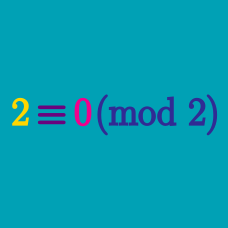Number Theory

# Modular Arithmetic - Exponentiation

As we've now covered modular addition and modular multiplication (repeated modular addition), the next operation to consider is repeated modular multiplication, aka modular exponentiation!

Given that $9 \equiv 2 \pmod{7},$ what is $9 \times 9 \times 9 \times 9 \times 9 \pmod{7}?$

What is $2 ^ {15} \pmod{11} ?$

What is $5 ^ {34} \pmod{31} ?$

Which of the following is congruent to $\large 7^{7^{7^{7^7}}} \pmod {10}?$

What is $2 ^ {7} \pmod{10} ?$

×